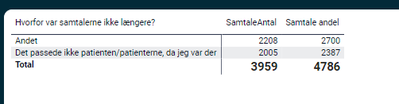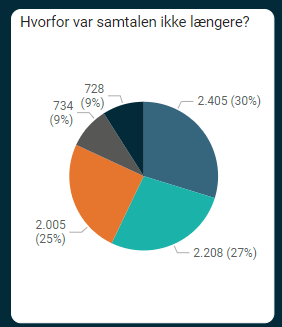cancel
Showing results for
Did you mean:Super User

## My total in my matrix is wrong? (Multiple questionare setup for Power BI)

My datamodel is a typical multiple questionare model with bothways joins for all the dimensions with one Fact having each surveyId once and then the dimensions can have the several answers for every question (the model is described in here several threads):I was asked to show percentage of total, for this question:Where 'Ukendt' (=Unknown) is removed. That's when the trouble started 😞

I normally would use this measure to create a grand total:

``````Diagnose andel = DIVIDE (
[Antal respondenter],

CALCULATE ( [Antal respondenter],  ALLSELECTED(DimDiagnose[Hvilken diagnose havde personen?]))
)``````

But the total is wrong:I removed other answers in the table, but the chart for the same answers show the same amount. But the total is wrong as can be seen. I can create this pie chart:And the percentage is correct, but I would prefer the stack chart and that I could calculate the total myself. What am I missing?

1 ACCEPTED SOLUTIONSuper User

Hi @Bokazoit ,

For this you need to create a measure that calculates the total value best option is that measure is a new one so you can easily debug and reuse it.

Try the following code:

``Total Value = SUMX(Values(Table[Hvorfor var samtalerne ikke laenger?]),[Your Metric])``

If you need this to be used in several metrics you must create a calculation item.

Regards

Miguel Félix

Proud to be a Super User!

Check out my blog: Power BI em Português2 REPLIES 2Super User

Hi @Bokazoit ,

For this you need to create a measure that calculates the total value best option is that measure is a new one so you can easily debug and reuse it.

Try the following code:

``Total Value = SUMX(Values(Table[Hvorfor var samtalerne ikke laenger?]),[Your Metric])``

If you need this to be used in several metrics you must create a calculation item.

Regards

Miguel Félix

Proud to be a Super User!

Check out my blog: Power BI em PortuguêsSuper User

Tx that did the trick 🙂

``````Total Value =
DIVIDE([Antal samtaler],
CALCULATE(
SUMX(Values('DimSamtaleLængde'[Hvorfor var samtalerne ikke længere?]),[Antal samtaler]),
ALLSELECTED('DimSamtaleLængde'[Hvorfor var samtalerne ikke længere?])
)
)``````Announcements#### Exclusive opportunity for Women!

Join us for a free, hands-on Microsoft workshop led by women trainers for women where you will learn how to build a Dashboard in a Day!#### Power Platform Conference-Power BI and Fabric Sessions

Join us Oct 1 - 6 in Las Vegas for the Microsoft Power Platform Conference.Top Solution Authors
Top Kudoed Authors
Users online (1,429)